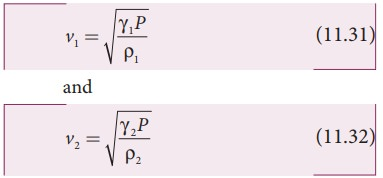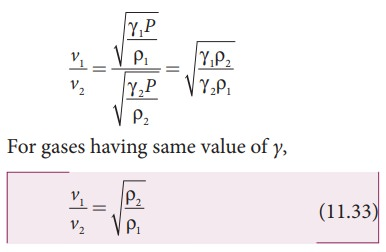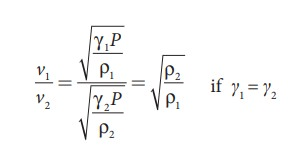Home | | Physics 11th std | Factors affecting speed of sound in gases

# Factors affecting speed of sound in gases

(a) Effect of pressure (b) Effect of temperature (c) Effect of density (d) Effect of moisture (humidity) (e) Effect of wind

Factors affecting speed of sound in gases

Let us consider an ideal gas whose equation of state iswhere, P is pressure, V is volume, T is temperature, n is number of mole and R is universal gas constant. For a given mass of a molecule, equation (11.26) can be written asFor a fixed mass m, density of the gas inversely varies with volume. i.e.,Substituting equation (11.28) in equation (11.27), we getwhere c is constant.

The speed of sound in air given in equation (11.25) can be written asFrom the above relation we observe the following

## (a) Effect of pressure :

For a fixed temperature, when the pressure varies, correspondingly density also varies such that the ratio (P/ρ) becomes constant.

This means that the speed of sound is independent of pressure for a fixed temperature. If the temperature remains same at the top and the bottom of a mountain then the speed of sound will remain same at these two points. But, in practice, the temperatures are not same at top and bottom of a mountain; hence, the speed of sound is different at different points.

## (b) Effect of temperature :the speed of sound varies directly to the square root of temperature in kelvin.

Let v0 be the speed of sound at temperature at 0° C or 273 K and v be the speed of sound at any arbitrary temperature T (in kelvin), thenSince v0 = 331m s-1 at 00C, v at any temperature in t0C is

= (331 + 0.60t) m s-1

Thus the speed of sound in air increases by 0.61 m s-1 per degree celcius rise in temperature. Note that when the temperature is increased, the molecules will vibrate faster due to gain in thermal energy and hence, speed of sound increases.

## (c) Effect of density :

Let us consider two gases with different densities having same temperature and pressure. Then the speed of sound in the two gases areTaking ratio of equation (11.31) and equation (11.32), we getThus the velocity of sound in a gas is inversely proportional to the square root of the density of the gas.

## (d) Effect of moisture (humidity):

We know that density of moist air is 0.625 of that of dry air, which means the presence of moisture in air (increase in humidity) decreases its density. Therefore, speed of sound increases with rise in humidity. From equation (11.30)Let ρ1v1 and ρ2v2 be the density and speeds of sound in dry air and moist air, respectively. ThenSince P is the total atmospheric pressure, it can be shown thatwhere p1 and p2 are the partial pressures of dry air and water vapour respectively. Then## (e) Effect of wind:

The speed of sound is also affected by blowing of wind. In the direction along the wind blowing, the speed of sound increases whereas in the direction opposite to wind blowing, the speed of sound decreases.

### EXAMPLE 11.9

The ratio of the densities of oxygen and nitrogen is 16:14. Calculate the temperature when the speed of sound in nitrogen gas at 17°C is equal to the speed of sound in oxygen gas.

### Solution

From equation (11.25), we haveWhere, R is the universal gas constant and M is the molecular mass of the gas. The speed of sound in nitrogen gas at 17°C isSimilarly, the speed of sound in oxygen gas at t in K isGiven that the value of γ is same for both the gases, the two speeds must be equal. Hence, equating equation (1) and (2), we getSquaring on both sides and cancelling γ R term and rearranging, we getSince the densities of oxygen and nitrogen is 16:14,Substituting equation (5) in equation (3), we getStudy Material, Lecturing Notes, Assignment, Reference, Wiki description explanation, brief detail
11th Physics : UNIT 11 : Waves : Factors affecting speed of sound in gases |